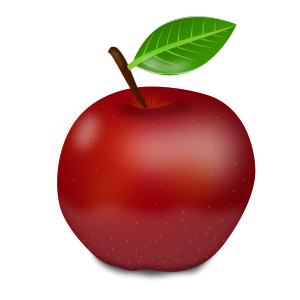# Input data

## In words...

Machine learning works from data. In supervised learning, the machine is given both input data and output labels, from which it produces a model that can predict the label for any input. In unsupervised learning, the machine is only given a set of input data from which it should extract knowledge.

The nature of the input depends directly on the application. For instance, if we want to predict if it is likely to rain in the next hour, the input should contain a representation of the meteorological variables influencing this fact.

Most machine learning algorithms (at least the ones discussed in this book) work with inputs that are vectors of a given dimension. In these vectors, each component corresponds to a feature of the input object/pattern represented by the vector.

Selecting the right features for a given problem is a complex task, that can be automated to some extend by feature selection methods. Also, dimensionality reduction methods aims at constructing a smaller set of new features such that they can approximately represent objects as well.

## In pictures...

We want to make a computer learn to distinguish between apples and bananas. To do this, we have to input data about a given fruit to the computer. There are many ways to do this. Consider a simple scheme where the user inputs the width and height of the fruit to the computer. Then, every possible fruit is represented in the computer memory by two numbers and in mathematical terms by a vector of dimension 2. Such vectors can be conveniently visualized as points in a 2D plot.

The plot on the right shows the data points corresponding to the fruits plotted on the left. You can click on fruits to see which data point they correspond to.

You can also add custom fruits to the plot:Apple width :
Apple height :

### Objects respresentations often lead to high-dimensional vectors

Instead of measuring the width and height of the fruit, we could also take a picture of the fruit and give it as input to the learning machine...An image can be fully described by the color of each pixel. A typical representation of a color is obtained via the amount of red, green and blue that are mixed to obtain the color. This means that an entire image can be represented by a collection of 3 numbers for each pixel, and thus by a vector of dimension 3 times the width times the height of the image (for instance, the image on the left corresponds to a vector of dimension $3 \times 200 \times 200 = 120\,000$).

If we look at a single row of the image, here row # (hover over the image to see all the different rows): it corresponds to 3 x 200 = 600 numbers (hover over the pixels in the row to highlight the 3 numbers corresponding to its red, green and blue components):

## In maths...

We denote an input by $\g x$ and assume that all inputs are taken from a set of possible inputs, known as the input space $\X$.

In this entire book, we restrain ourselves to inputs that are real $d$-dimensional vectors, i.e., $$\g x \in \X\subseteq \R^d .$$ The word features will refer to the components $x_j\in\R^d$, $j=1,\dots,d$, of the input vectors $\g x= [x_1,\dots, x_d]^T$.
We will often consider sets of input vectors indexed by $i$ as $\g x_i$. In this case, the $j$th component of $\g x_i$ is denoted $x_{ij}$.

Most of the time, we will also consider inputs as random variables $X$ taking values in $\R^d$. In this context, $\g x$ refers to a realization of $X$.